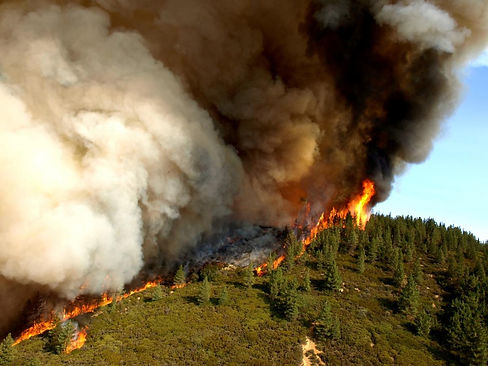top of page

## PROJECTS###### Optimal Control of Propagation Fronts

We consider a controlled reaction-diffusion equation, motivated by a pest eradication problem. Our goal is to derive a simpler model, describing the controlled evolution of a contaminated set. In this direction, the first part of the paper studies the optimal control of 1-dimensional traveling wave profiles. Using Stokes' formula, explicit solutions are obtained, which in some cases require measure-valued optimal controls. In the last section we introduce a family of optimization problems for a moving set. We show how these can be derived from the original parabolic problems, by taking a sharp interface limit.

This research is available as a paper here.

Image courtesy of Wikipedia.###### Optimal Control of Moving Sets

Motivated by the control of invasive biological populations, we consider a class of optimization problems for moving sets

t↦ Ω (t)⊂ IR2. Given an initial set Ω0, the goal is to minimize the area of the contaminated set Ω(t) over time, plus a cost related to the control effort. Here the control function is the inward normal speed along the boundary ∂Ω(t). We prove the existence of optimal solutions, within a class of sets with finite perimeter. Necessary conditions for optimality are then derived, in the form of a Pontryagin maximum principle. Additional optimality conditions show that the sets Ω(t) cannot have certain types of outward or inward corners. Finally, some explicit solutions are presented.

The detailed results are available here.

Image courtesy of Sven Torfinn.